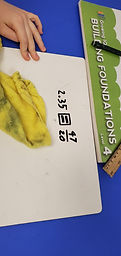Mr. Jake

Target 1​

Lesson Type:

New

Number Operation

:

Number Reltionships

Compare numbers, recording the results of the comparison with the symbol >, =, and <.

1:

Compare rational numbers (decimals and fractions).

2:

Compare fractions (proper and improper).

3:

Compare decimals (up to the hundredths place).

4:

When comparing decimals, understand to look at the greatest place-value first.

6th

Vocabulary:

Fraction, Decimal, Comparison

Activities:

Compared fractional and decimal values using inequality symbolsHome Exploration

Guiding Questions:Absent Students:

Target 2

:

1:

Construct and read pie (circle) graphs.

2:

Define circle graph.

3:

Identify the parts of a circle graph.

4:

Recognize that a circle graph provides a visual representation of the whole and its parts.

5:

Determine the fraction that each part of the circle graph represents.

7th

Vocabulary:

Circle Graph, Pie Chart, Fraction, Data

Activities:

Collected data to create pie charts

Answered questions based on information given in pie chartsHome Exploration

Guiding Questions:Target 3

:

Vocabulary:

Activities:Home Exploration